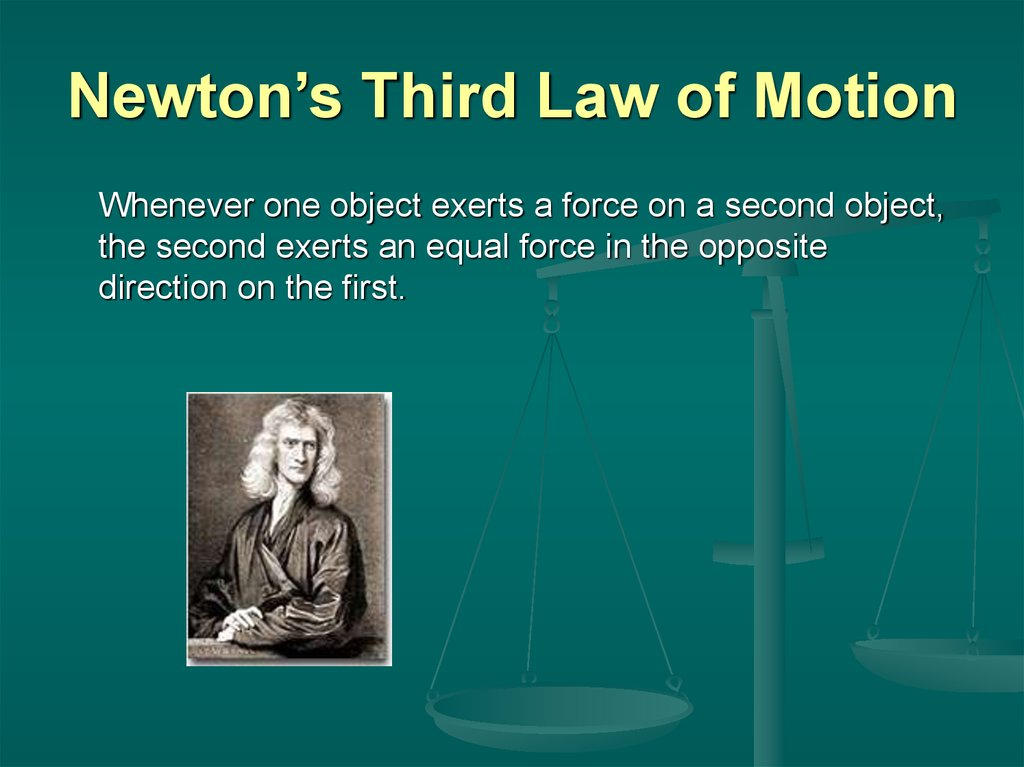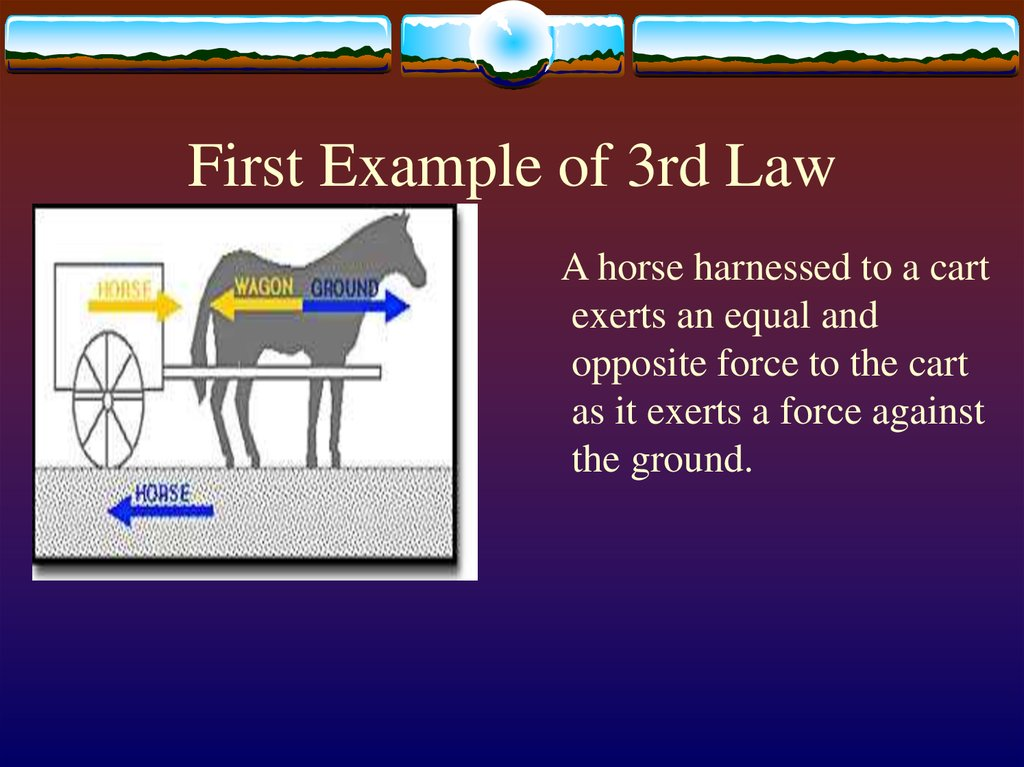# Newton’s Third Law of Motion

## 1. Newton’s Third Law of Motion

Whenever one object exerts a force on a second object,
the second exerts an equal force in the opposite
direction on the first.

## 2. First Example of 3rd Law

A horse harnessed to a cart
exerts an equal and
opposite force to the cart
as it exerts a force against
the ground.

## 3. Second Example of 3rd Law

Space shuttle’s
rocket boosters
propel the orbiter
into space by
exerting an equal
and opposite force
to exhaust gasses.

## 4. Concept Question 1

Why are we able to
walk?

Force exerted
on the person’s
foot by the
ground. Fpg
Fgp=-Fpg
We walk forward
because when one
foot pushes
backward against
the ground, the
ground pushes
forward on that foot.
Force exerted on the ground
by person’s foot. Fgp

## 6. Concept Question 2

What makes a car
go forward?

By Newton’s third law,
the ground pushes on
the tires in the
opposite direction,
accelerating the car
forward.

## 8. Concept Question

Which is stronger,
the Earth’s pull on
an orbiting space
shuttle or the space
shuttle’s pull on the
earth?

According to Newton’s Third
Law, the two forces are
equal and opposite. Because
of the huge difference in
masses, however the space
shuttle accelerates much
more towards the Earth than
the Earth accelerates toward
the space shuttle.
a = F/m

## 10. Problem 1

What force is needed to
accelerate the 60 kg
cart at 2m/s^2?

## 11. How to solve Problem 1

What force is needed to accelerate the
60kg cart at 2 m/s^2?
Force = mass times
acceleration
F=m*a
F = 60kg * 2m/s^2
F = 120kgm/s^2
Kgm/s^2 = Newton
Newton = N
F =120 N

## 12. Problem 2

A force of 200 N
accelerates a bike and
rider at 2 m/s^2. What
is the mass of the bike
and rider?

## 13. How to solve Problem 2

A force of 200 N accelerate a bike
and rider at 2m/s^2. What is the
mass of the bike and rider?
F = ma therefore:m =F/a
m = 200N/2m/s^2
N= kgm/s^2 so when divide